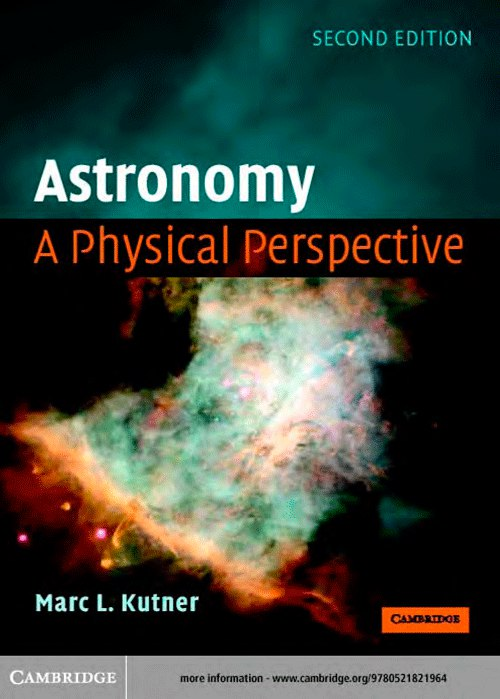# Astronomy: A Physical PerspectiveАвторы: Kutner M.
Издательство: Cambridge University Press
Форматы: pdf
Описание: This revised and updated comprehensive introduction to astronomical objects and phenomena applies basic physical principles to a variety of situations. Students learn how to relate everyday physics to the astronomical world with the help of useful equations, chapter summaries, worked examples and end-of-chapter problem sets. It will be suitable for undergraduate students taking a first course in astronomy, and assumes a basic knowledge of physics with calculus.